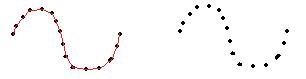# How to create a linear and quadratic function and graph them

hello forum

I am new to Snap and would like to know how I can create a linear and quadratic math function and then graph them.
I must assign variables x through a table of values ​​to obtain the values ​​of the equation represented by y, and then I must graph both functions.

Can you help me if there is a tutorial on this.

Thank you

do you know how to do linear and quadratic functions in math, and how they're graphed?

this sounds suspiciously like a homework question, we don't do homework for people here.

Yes of course I know that. I would do the linear function first and then the quadratic, of course I would do it separately.

If I know how to do a linear or quadratic function

The subject is the management of the blocks, which is the block that graphs the function? and with which block can I load a table of values ​​for x? That's what I'm asking. Thanks.

i think that i have a par abolas projet and you can dissect some quadratics and logithmitics

Thanks d4s_over_dt4. If you have some projects where it helps me to calculate a linear or quadratic function with tables of values, it can help me a lot. If you want I hope that project. Thanks in advance.

for graphing, you can use the position on the stage, and the pen to draw on the stage.

There isn't a GRAPH block that does it all for you. Your job is to write one!

One easy way to get a table of values is the block NUMBERS FROM __ TO __.

I think we have to know a little more about you to be able to give you good advice. Are you new to block programming? Have you programmed in Scratch?

i'm pretty sure scisnap actually has graphing blocks, but they're pretty cumbersome and it would probably be a better to learn to write one

Oh I'm sure it would do his entire homework for him! :~)

Hello bh. if I am new to block programming. Anyway, I already got the graphics in Scratch and also in Snap. Finding out I was doing it. in both tools. I already did. Thank you.

For graphing, you can use motion blocks like set x and y, and control blocks like repeat and also pencil blocks to draw on the stage like stamp. I already got the graph. Thank you and keep going. Thank you ego-lay_atman-bay

I already got the line graph. If with the blocks that exist I already did it, such as movement blocks such as fix x, fix y, control how to repeat and pencil blocks such as stamp. Thanks sarpnt.To do picture 1:

goto 1st point
pen down
for  each point in the list
goto the point
pen up


To do picture 2:

pen down
for each point in the list
pen up
go to the point
pen down
move (0)        (this will draw the point)
pen up


I think the idea is that he doesn't have a list of points, just a function.

Linear functions are typically in the form y = mx + b and are graphed as straight lines. To draw a linear graph, start with the y-intercept or b value, then use the slope to find a second point. Quadratic functions are typically in the form y = ax2 + bx + c and are graphed as curved parabolas.

This topic was automatically closed 30 days after the last reply. New replies are no longer allowed.